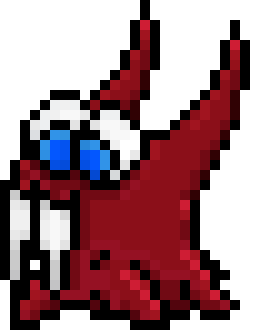November 13, 2019, 05:28:43 am

## News:

Juju thinks he's so clever by putting funny stuff here

You can help CodeWalrus stay online by donating here.

## PolyJS

Started by _iPhoenix_, May 19, 2018, 05:55:57 am

0 Members and 1 Guest are viewing this topic.

#### _iPhoenix_

#####May 19, 2018, 05:55:57 amLast Edit: May 19, 2018, 06:12:53 am by _iPhoenix_
PolyJS

PolyJS is a lightweight, fast, and easy to use JavaScript library for manipulating 2D polygons on the HTML5 Canvas.

It lets you create, modify and draw polygons. It also lets you attach points to other points (with or without an offset) so that you can potentially change everything on the screen just by adjusting one value.

I really want to see what kinds of games and art people can make using PolyJS. It lets you do this unique, simple, and interesting art style.

You can download the docs from here, and the latest build from here! Happy Polygoning!

(If you are interested in contributing or just want to poke around, my GitHub repo is here)"walruses are better than tuxedo chickens, all hail the great" ~ me
Evolution of my avatar:#### _iPhoenix_

#####May 24, 2018, 09:58:05 pm #1
*bump*I just released v0.2.0!

This adds several new features, including the ability to change the edge thickness, get the coords of a point (some points may not explicitly say what their coords are), and the ability to use the string "self" when fixing points to other points on the same Polygon object.

You can get the revised build from here and the docs from here."walruses are better than tuxedo chickens, all hail the great" ~ me
Evolution of my avatar:#### _iPhoenix_

#####May 25, 2018, 07:04:09 am #2
I forgot to post my demos! I think this deserves a double post, because they are coolHere's  a demo. The shapes do not have to be triangles!`var pJS = new PolyJS(document.getElementById("canvas"));pJS.canvas.width = 240;pJS.canvas.height = 240;function createTriMesh(width, height, triWidth, triHeight, skewFactor) {  var index = -1,    horizTris = width / triWidth;  var skewX = () => (Math.floor(Math.random() * 2) - 1) * Math.floor((triWidth * Math.random()) / skewFactor),    skewY = () => (Math.floor(Math.random() * 2) - 1) * Math.floor((triHeight * Math.random()) / skewFactor)  for (var j = 0; j < height; j += triHeight) {    for (var i = 0; i < width; i += triWidth) {      var a = i / triWidth,        b = j / triHeight;      index++;      var points = [        [i + skewX(), j + triHeight + skewY()]      ];      if (i) {        points = {          attached: [pJS.polygons[pJS.polygons.length - 1], 1]        };      }      if (j) {        points.push({          attached: [pJS.polygons[2 * ((b - 1) * horizTris + a)], 0]        });        points.push({          attached: [pJS.polygons[2 * ((b - 1) * horizTris + a) + 1], 1]        });      } else {        points.push([i, j]);        points.push([i + triWidth, j]);      }      var lastPolygon = new pJS.Polygon(points, color(), color());      new pJS.Polygon([{          attached: [lastPolygon, 2]        },        [i + triWidth + skewX(), j + triHeight + skewY()], {          attached: [lastPolygon, 0]        }      ], color(), color());    }  }  pJS.draw()}function color() {  return "#" + Math.floor(256 * Math.random()).toString(16).padStart(2, 0) + Math.floor(256 * Math.random()).toString(16).padStart(2, 0) + Math.floor(256 * Math.random()).toString(16).padStart(2, 0)}createTriMesh(240, 240, 30, 30, 2.5);`
Please ignore the low code quality; that demo was made in less than 15 minutes without any planning... x.x

Here's a much more simple example that I posted in SAX/IRC about a week or two ago. It draws two triangles and alters them after a short delay, and it explains how it works better, too.
`//create a <canvas> element and add it to the page.var canvas = document.createElement("canvas");document.body.appendChild(canvas);//initialize a PolyJS object with the canvasvar pJS = new PolyJS(canvas);//create a Polygonvar shape1 = new pJS.Polygon([  [0, 0],  [0, 100],  [100, 0]], "black", "green", true);//create another Polygonvar shape2 = new pJS.Polygon([{    attached: [pJS.polygons, 1] //attached to the first point in the first Polygon created.  }, {    attached: [pJS.polygons, 2]  }, //attached to the 3rd point in the first Polygon created  [100, 50]], "black", "red", true);pJS.draw();//after a delay of 1/2 second, change two points in the first shape and redraw.setTimeout(x => {  var points = shape1.points;  shape1.setPoint(1, [0, 20]);  shape1.setPoint(2, [128, 32]);  pJS.draw(true);}, 500);`"walruses are better than tuxedo chickens, all hail the great" ~ me